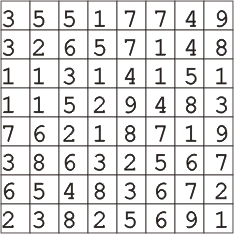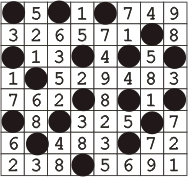Quickly add a free MyWikiBiz directory listing!

# Punzhu Puzzles: Hitori

Hitori

Hitori puzzles were first published in Japan by Nikoli and are a logic puzzle similar somewhat to sudoku.

The puzzle is based on a grid in which numbers are placed in each square. To solve a Hitori puzzle a player must cross out or circle squares so that: 1) no number appears more than once in each row or column, 2) crossed out or circled squares are not adjacent and, 3) all the remaining squares, two squares or more, allow you to move horizontally or vertically without hitting a crossed out or circled square.

To design a Hitori puzzle we first set the number of squares we want across and down. This is the grid. Then we input the numbers that become the puzzle. We do this manually, as compared to some Hitori puzzles that are computer generated and don't always follow proper technique. In some computer generated puzzles for example, two adjacent squares will be created and is thus a false Hitori.

Punzhu Puzzles was the first publisher of symbol Hitori puzzles in a puzzle magazine. Symbol Hitori puzzles use symbols instead of numbers and thereby introduce a new level of concentration to the game.

Here are examples of a grid and a solution to a standard non-symbol Punzhu Puzzles Hitori puzzle:Free Puzzle Magazines